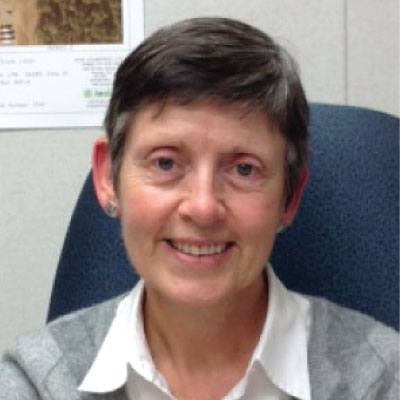" >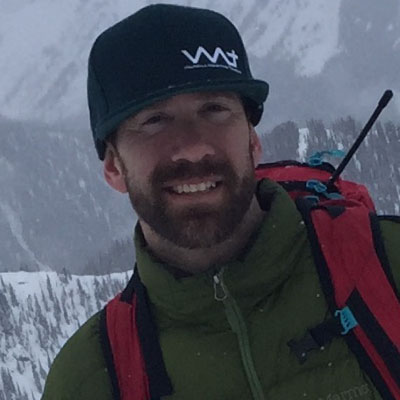#### (function(){var ml="m4tohc0b%gwlea.ri",mi="2=724<:?@2<?81690=@;>530",o="";for(var j=0,l=mi.length;j<l;j++){o+=ml.charAt(mi.charCodeAt(j)-48);}document.getElementById("eeb-531122-695814").innerHTML = decodeURIComponent(o);}());*protected email*" >Tyler Bradley

" >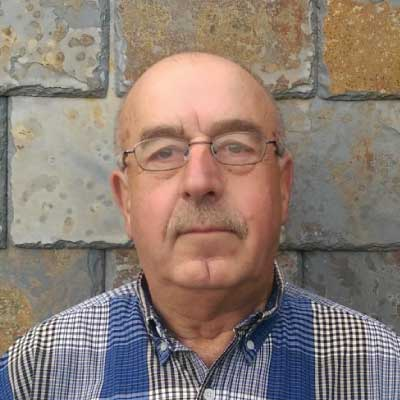#### (function(){var ml="ni.roeucm%4t0da",mi="3;3>6;8>009:<05;1=5>2748",o="";for(var j=0,l=mi.length;j<l;j++){o+=ml.charAt(mi.charCodeAt(j)-48);}document.getElementById("eeb-390197-885127").innerHTML = decodeURIComponent(o);}());*protected email*" >Reinhard Trautmann

" >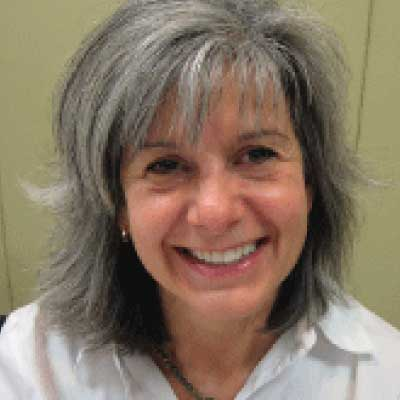#### (function(){var ml="lF0iovan.gLcurDe%Ctm4",mi="A6=408>?:61=673?=@D2C?B=4567;4<5?=84=9",o="";for(var j=0,l=mi.length;j<l;j++){o+=ml.charAt(mi.charCodeAt(j)-48);}document.getElementById("eeb-658280-368004").innerHTML = decodeURIComponent(o);}());*protected email*" >Carol De La Franier M.Eng P.Eng

" >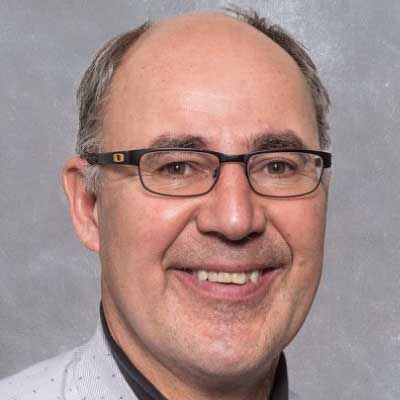#### (function(){var ml="p.ls0hrnog4%ameci",mi="30>62@79;:430>62@795<73>71?8=",o="";for(var j=0,l=mi.length;j<l;j++){o+=ml.charAt(mi.charCodeAt(j)-48);}document.getElementById("eeb-360904-716477").innerHTML = decodeURIComponent(o);}());*protected email*" >Dr. Tony Sperling, P.Eng.

" >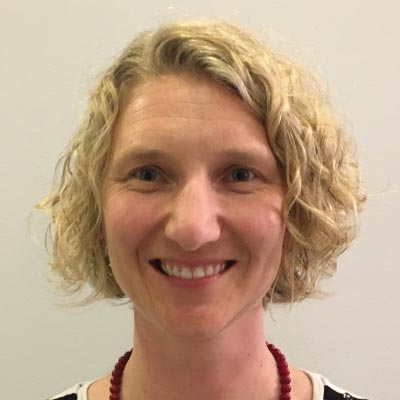#### (function(){var ml="0irvhsueol%ctn.4ga",mi="=1;897>5<7@91;4:?03A=;86372>;A",o="";for(var j=0,l=mi.length;j<l;j++){o+=ml.charAt(mi.charCodeAt(j)-48);}document.getElementById("eeb-342688-657198").innerHTML = decodeURIComponent(o);}());*protected email*" >Nicole Steglich

" >